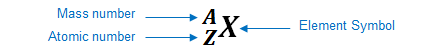# Problem: Determine the isotope symbol that fits each description. a. 68 neutrons, 47 electrons b. mass number = 197, 79 electrons c. atomic number = 86, 136 neutrons d. atomic number = 76, mass number = 192

###### FREE Expert Solutiona. 68 neutrons, 47 electrons

neutral atom: atomic number = # of protons = # of electrons

# of protons = 47

atomic number (Z) = 47
atomic # 47 → silver (Ag)

mass number = # of neutrons + # of protons
mass number (A) = 68 + 47
mass number (A) = 115

Isotope symbol

$\overline{){}_{\mathbf{47}}{}^{\mathbf{115}}\mathbf{Ag}}$

b. mass number = 197, 79 electrons

# of protons = 79

atomic number (Z) = 79
atomic # 79 → gold (Au)

84% (144 ratings)###### Problem Details

Determine the isotope symbol that fits each description.

a. 68 neutrons, 47 electrons

b. mass number = 197, 79 electrons

c. atomic number = 86, 136 neutrons

d. atomic number = 76, mass number = 192